Courses

# Test: XAT Quant 2016

## 29 Questions MCQ Test Quantitative Aptitude (Quant) | Test: XAT Quant 2016

Description
This mock test of Test: XAT Quant 2016 for CAT helps you for every CAT entrance exam. This contains 29 Multiple Choice Questions for CAT Test: XAT Quant 2016 (mcq) to study with solutions a complete question bank. The solved questions answers in this Test: XAT Quant 2016 quiz give you a good mix of easy questions and tough questions. CAT students definitely take this Test: XAT Quant 2016 exercise for a better result in the exam. You can find other Test: XAT Quant 2016 extra questions, long questions & short questions for CAT on EduRev as well by searching above.
QUESTION: 1

### Consider the set of numbers {1, 3, 32, 33... 3100}. The ratio of the last number and the sum of the remaining numbers is closest to:

Solution: Sum of the remaining numbers would be a GP series whosea = 1, n = 100 and r = 3.

Thus, ratio would be (3100/ [(1 x 3100 - 1)/3-1]) = 2

We are assuming (for all practical purposes) 1 x 3100 – 1 = 3100

QUESTION: 2

### Anita, Biplove, Cheryl, Danish, Emily and Feroze compared their marks among themselves.Anita scored the highest marks, Biplove scored more than Danish. Cheryl scored more than at least two others and Emily had not scored the lowest.Statement I: Exactly two members scored less than Cheryl.Statement II: Emily and Feroze scored the same marks.Which of the following statements would be sufficient to identify the one with the lowestmarks?

Solution: From the main part we get

A is highest.

B > D.

E is not at 6th position.

C can have positions 2, 3 or 4.

Hence, 6th position holder can be D or F.

From Statement I: C is at 4th position.

Using Statement I we cannot confirm 6th position holder. It can be D or F.

Form statement II: E = F. As E is not in 6th position, F is also not in 6th position.

Then, D is 6th position holder.

Option B is the correct answer.

QUESTION: 3

### Rani bought more apples than oranges. She sells apples at Rs. 23 apiece and makes 15%profit. She sells oranges at Rs. 10 apiece and marks 25% profit. If she gets Rs. 653 afterselling all the apples and oranges, find her profit percentage.

Solution: Assume Rani sells X apples and Y oranges, where X > Y

Then, 23X + 10Y = 653

Then try X = 1, 11, 21 etc. (to get last digit = 3)

We find X = 21 and Y = 17 is the only solution where X > Y

Cost of an Apple = 23/1.15 and cost of an Orange = 10/1.25

Overall cost = 483/1.15 + 170/1.25 = 556

Then profit = 653 – 556 = 97

Profit percentage = (97/556) x 100 = 17.4%

QUESTION: 4

In an amusement park along with the entry pass a visitor gets two of the three availablerides (A, B and C) free. ON a particular day 77 opted for ride A, 55 opted for B and 50 opted for C; 25 visitors opted for both A and C, 22 opted for both A and B, while no visitor opted for both B and C. 40 visitors did not opt for ride A and B, or both. How many visited with the entry pass on that day?

Solution: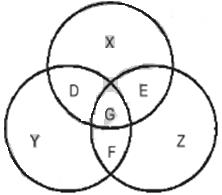Assume, M = Number of visitors who did not opt for any of A, B or C.

Given,

X + D + E + G = 77

Y + D + G + F = 55

Z + E + G + F = 50

Z + M = 40

E + G = 25

D + G = 22

G + F = 0

Then, G = 0, F = 0.

E = 25

D = 22

X = 30

Y = 33

Z = 25

M = 15

Total number of visitors = X + Y + Z + D + E + F + G + M = 150

Hence, Option E is the correct answer.

QUESTION: 5

ΔABC and ΔXYZ are equilateral triangles of 54 cm sides. All smaller triangles like ΔANM, ΔOCP, ΔQPX etc. are also equilateral triangles. Find the area of the shape MNOPQRM.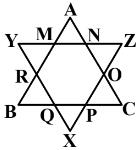Solution:

Area of any equilateral triangle = (√3/2) x (side) 4AB = 54 cm. Then, MR = 18 cm. Area of ∆YMR =81√3 sq. cm. and

area of ∆XYZ = 729√3 sq. cm.

Finally, area of shape MNOPQRM

= 729√3 – 3 x 81√3 = 486√3 sq. cm.

Using formula of hexagon, area of shape MNOPQRM

= (3√3/2) x 182 = 486 √3 sq. cm.

QUESTION: 6

In the figure below, AB = AC = CD. If < />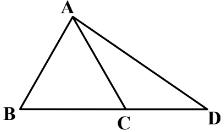Solution: Given, AB = AC. Then ∠ABC =∠ACB

From the properties of triangle,

Now, ∠BAC = 180°−∠ABC −∠ACB

= 180°− 40°− 40°= 100°

Hence, Option D is the correct answer.

QUESTION: 7

Akhtar plans to cover a rectangular floor of dimensions 9.5 meters and 11.5 meters using tiles. Two types of square shaped tiles are available in the market. A tile with side 1-metercosts Rs. 100 and a tile with side 0.5 meters costs Rs. 30. The tiles can be cut if required.What will be the minimum cost of covering the entire floor with tiles?

Solution: We should use 1 meter tiles (cost 100) before 0.5 meter side tiles (cost 4 × 30 = 120).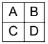Area of A = 9 × 11 sq. meters

Area of B = 0.5 × 11 sq. meters

Area of C = 0.5 × 9 sq. meters

Area of D = 0.5 × 0.5 sq. meters

For A we need 99 tiles with 1 meter side

For B and C together we need (11 + 9)/2 tiles with 1 meter side

(we need to break each tile into two equal areas)

For D we need 1 tile with 0.5 meter side

Hence, the total cost = 9900 + 1000 + 30 = 10930

QUESTION: 8

Pradeep could either walk or drive to office. The time taken to walk to the office is 8 timesthe driving time. One day, his wife took the car making him walk to office. After walking 1 km, he reached a temple when his wife called to say that he can now take the car. Pradeep figure that continuing to walk to the office will take as long as walking back home and then driving to the office. Calculate the distance between the temple and the office.

Solution: Suppose the distance between his home and office is x km.

Then, distance between his office and temple is (x – 1) km.

If he walks at a speed of y km/hr. Then his driving speed

= 8y km/hour, as he is 8 times faster in car.

Then,

1/y + x/8y = (x - 1)/y

or, 1+ x/8y = x-1

or, x = 16/7

finally, x – 1 = 9/7

Option C is the correct answer.

QUESTION: 9

Each day on Planet M is 10 hours, each hour 60 minutes and each minute 40 seconds. Theinhabitants of Planet M use 10-hour analog clock with an hour hand, a minute hand and a second hand. If one such clock shows 3 hours 42 minutes and 20 seconds in a mirror what will be the time in Planet M exactly after 5 minutes?

Solution: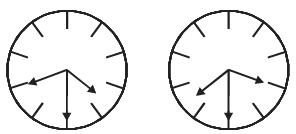In the above figure, left side diagram shows mirror image and right side diagram shows actual clock.

Form the drawing, actual time = 6 hours 17 min 20 seconds.

After 5 minutes actual time = 6 hours 22 min 20 seconds.

Option B is the correct answer.

QUESTION: 10

A square piece of paper is folded three times along its diagonal to get an isosceles trianglewhose equal sides are 10 cm. What is the area of the unfolder original piece of paper?

Solution: The smaller side of the isosceles triangle = 10

Then the side of the square = 20 and the area of the square

= 400 sq. cm.

Option A is the correct answer.

QUESTION: 11

The difference between the area of the circumscribed circle and the area of the inscribedcircle of an equilateral triangle is 2156 sq. cm. What is the area of the equilateral triangle?

Solution:

In an equilateral triangle, both circles have same centre (point B). Assume, AB is radius of circumscribed circle and BC is the radius of inscribed circle, where BCA is right angle. Then,

BC/AB = sin30° = ½

Let, BC = x and AB = 2x.

Then, AC = x√3

Side of the triangle = 2x√3

Area of the triangle = 12x2√3 /4

Then, 4πx2 – πx2 = 2156 Or x2 = 2156 x 7/66

Finally, area of the triangle = 686√3

Option A is the correct answer.

QUESTION: 12

A person standing on the ground at point A saw an object at point B on the ground at adistance of 600 meters. The object started flying towards him at an angle of 30° with theground. The person saw the object for the second time at point C flying at 30° angle withhim. At point C, the object changed direction and continued flying upwards. The person sawthe object for the third time when the object was directly above him. The object was flyingat a constant speed of 10 kmph.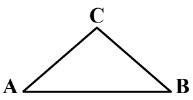Find the angle at which the object was flying after the person saw it for the second time. You may use additional statement(s) if required.

Statement I: After changing direction the object took 3 more minutes than it had taken before.

Statement II: After changing direction the object travelled an additional 200√3 meters.

Which of the following is the correct option?

Solution: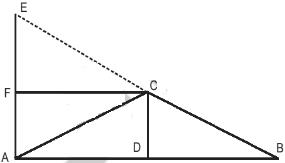Given, AB = 600 meters. ∠ CAE =° 60. Assume D is the middle point of AB. Then AD = 300 meters.

As ∠CAB =∠CBA, CA = CB and ∠ACD is right angled triangle.

Also, AD/AC = cos30° Then, AC = 600/√3 = 200√3 = CB

From Statement I:

CE = 500 meters. Assume F is perpendicular on AE.

Then, ∠ACF = 30° and ∠ CAF = 60°. Also, CF = 300 meters.

Then, cos ∠ECF = 300/500 = 3/5. So, we can get the value of

∠ECF, the new angle.

From Statement II: CE = 500 meters = CA. Then,

∠ECF = 30°, which is different from the angle found usingStatement I.

Hence, Option D is the correct answer.

QUESTION: 13

f is a function for which f (1) = 1 and f(x) = 2x + f(x – 1) for each natural number x ≥ 2. Find f (31)

Solution: f (31) = f (30) + 2×31 = f (29) + 2(31 + 30) = f (28) + 2(31 + 30 + 29) = ... = f (2) + 2(31 + 30 + 29 + ... + 3) = 2(31 + 30 + 29 + 3 + 2) +1

= 2 × 32 × 31/2 – 1 = 991

QUESTION: 14

Two numbers in the base system B are 2061B and 601B. The sum of these two numbers indecimal system is 432. Find the value of 1010B in decimal system.

Solution: In decimal system the values are

2061B = 2B3 + 6B + 1 and 601B = 6B2 + 1

Then, 2B3 + 6B2 + 6B + 2 = 432

Or, (B + 1)3 = 216 = 63

Hence, B = 5

Then, 1010B = B3 + B = 53 + 5 = 130

Option C is the correct answer.

QUESTION: 15

A water tank has M inlet pipes and N outlet pipes. An inlet pipe can fill the tank in 8 hourswhile an outlet pipe can empty the full tank in 12 hours. If all pipes are left open simultaneously, it takes 6 hours to fill the empty tank. What is the relationship between M and N?

Solution: M inlet pipes can fill the Mth/8 part of the tank in 1 hour and N outlet pipes can empty Nth/12 part of the tank in 1 hour. If all pipes are left open, in 1 hour the tank will be filled = M/8 – N/12 = 1/6 (the tank will take 6 hours to fill).

Then, 6M – 4N = 8

M = (8 + 4N)/6

There will be infinite number of solutions (N = 1, 4, 7, 10, 13, 16 ...) but none of the above ratios are correct. For example, above equation is solvable when M = 2, N = 1 or M = 4, N = 4. But the ratios 2 : 1 (i.e. M = 2, N = 1) or 1 : 1 (M = 4, N = 4) are not correct because the solution of the above equation will not be an integer for values like M = 4, N = 2 or M = 6, N = 6.

Therefore, Option E is the correct answer.

QUESTION: 16

Company ABC starts an educational program in collaboration with Institute XYZ. As per theagreement, ABC and XYZ will share profit in 60: 40 ratio. The initial investment of Rs. 100,000 on infrastructure is borne entirely by ABC whereas the running cost of Rs. 400 per student is borne by XYZ. If each student pays Rs. 200 for the program find the minimumnumber of students required to make the program profitable, assuming ABC wants to recover its investment in the very first year and the program has no seat limits.

Solution: For program to be profitable both companies must recover costs before they can start making profits.

Since, ABC wants to recoup investment in the first year and there is no limit of number of students profits can only be shared after both companies can reach a situation of minimum profits (zero profit), which would be: 400x + 100000 = 2000x,

Left hand side represents total costs and right hand side represents revenues. Let x be minimum number of students required to reach a situation of minimum profits (in this case 0).

For the entire program, the MINIMUM students required for minimum profit is = 100000/ (2000 - 400) = 62.5 = 63.

QUESTION: 17

For two positive integers a and b, if (a + b) (a + b) is divisible by 500, then the least possiblevalue of a × b is:

Solution: 500 can be written as 5 × 5 × 5 × 2 × 2. As the number is divisible by 500, (a + b) should be divisible by 10.

Then, least possible value of (a + b) = 10

Hence, least possible value of a×b = 9×1 = 9 (when a = 9 and b = 1)

Option B is the correct answer.

QUESTION: 18

Study the figure below and answer the question: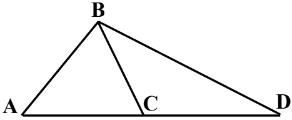Four persons walk from Point A to Point D following different routes. The one following ABCD takes 70 minutes. Another person takes 45 minutes following ABD. The third person takes 30 minutes following route ACD. The last person takes 65 minutes following route ABCD. IF all were to walk at the same speed, how long will it take to go from point B to point C?

Solution: AB + BC + CD = 70

AB + BD = 45

AC + CD = 30

AC + CB + BD = 65

Then, (AB + BC + CD) + (AC + CB + BD) = 70 +65 = 135

or, (AB + BD) + (AC + CD) + 2BC = 135

Finally, BC = 30

Option C is the correct answer.

QUESTION: 19

a, b, c are integers, |a| ≠ |b| ≠|c| and –10 ≤ a, b, c ≤ 10. What will be the maximum possible value of [abc – (a + b + c)]?

Solution: For maximum value, two of a, b, and c should be negative (all three negative will make abc negative). Hence the maximum will occur when a = –10, b = –9, and c = 8.

Then, abc = 731

Option C is the correct answer.

QUESTION: 20

ABCD is a quadrilateral such that AD = 9 cm, BC = 13 cm and ⎿DAB = ⎿BCD = 90°. P and Qare two points on AB and CD respectively, such that DQ: BP = 1: 2 and DQ is an integer. How many values can DQ take, for which the maximum possible area of the quadrilateralPBQD is 150 sq.cm?

Solution: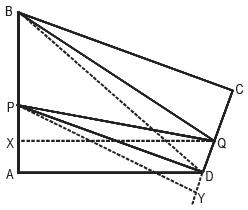Let’s assume DQ = x, CQ =y, AP = z. Thus, BP = 2x

Area of PBQD = Total area of the quadrilateral ABCD – (Area of triangle APD + Area of triangle BCQ)

Total area of the quadrilateral ABCD = Area of triangle ABD + Area of triangle BCD

Thus, Area of PBQD

[1/2 x (2x + z) x 9 + ½ x 13 x (x+y)] – [1/2 x 9 x z + ½ x 13 x y]

= 31x/2

According to the question, 31x/2 ≤ 150 ≤ or, x 9.68

Therefore, x can take 9 integer values. Option D is the correct answer.

QUESTION: 21

If a, b and c are 3 consecutive integers between –10 to +10 (both inclusive), how manyinteger values are possible for the expression (a3 + b3 + c3 + 3abc)/ (a+b+c) 2?

Solution: a3 + b3 + c3 – 3abc = (a + b + c)1/2 {(a – b)2 + (b – c)2 + (c –a)2}

For three consecutive integers, (x – 1), x and (x + 1), {(a – b)2 + (b – c)2 + (c – a)2} = 6, (a + b + c) = 3x and abc = x3 – x.

Then, (a3 + b3 + c3 + 3abc)/ (a+b+c) 2 = (a + b + c) 3 - 3abc + 6abc/ (a+b+c) 2

= (9x + 6x3 – 6x) / 9x2 = 1/3x + 2x/3

For the expression integer value is possible only if x = 1 and x = –1.

Possible values of a, b, c are (0, 1, 2) and (–2, –1, 0).

Hence, Option C is the correct answer.

QUESTION: 22

In the figure below, two circular curves create 60° and 90° angles with their respective centres. If the length of the bottom curve Y is 10π, find the length of the other curve.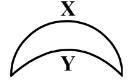Solution: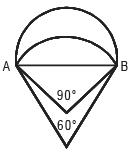Here, curve X creates 90° and curve Y creates 60°.

Take, radius of curve X = R1 and radius of curve Y = R2

For curve Y, 2πR2= 10π× 6.

Then, R2 = 30 = line AB.

Then, R1 = 15√2 Finally, length of curve X = 2πR2/ 4 = 15π / √2

Option A is the correct answer.

QUESTION: 23

Study the graph below and answer the questions that follow:

This graph depicts the last eight years’ annual salaries (in Rs. lacs.) offered to student during campus placement. Every year 100 students go through placement process. However, at least one of them fails to get placed. The salaries of all unplaced students are marked zero and represented in the graph.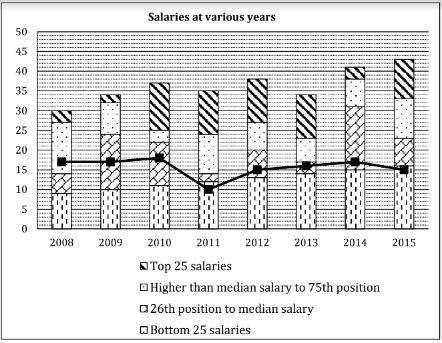The bold line in the graph presents Mean salaries at various years.

Q. In which year were a maximum number of students offered salaries between Rs. 20 to Rs. 30 lacs(both inclusive)?

Solution: At least 25% of the students definitely got salaries between 20-30 lacs. in years 2008, 2010 and 2012. However, the graphs do not tell the actual salaries. Hence, no comment can be made on the number of students within a particular salary range.

Option E is the correct answer.

QUESTION: 24

Study the graph below and answer the questions that follow:

This graph depicts the last eight years’ annual salaries (in Rs. lacs.) offered to student during campus placement. Every year 100 students go through placement process. However, at least one of them fails to get placed. The salaries of all unplaced students are marked zero and represented in the graph.The bold line in the graph presents Mean salaries at various years.

Q. Identify the years in which the annual median salary is higher by at least 60% than the average salary of the preceding year?

Solution: It is clear from the above table that in 2012 and 2014, the median of existing year is higher than 1.6 times mean of previous years. Hence the correct answer is: 2012 and 2014.

Option B is the correct answer.

QUESTION: 25

Study the graph below and answer the questions that follow:

This graph depicts the last eight years’ annual salaries (in Rs. lacs.) offered to student during campus placement. Every year 100 students go through placement process. However, at least one of them fails to get placed. The salaries of all unplaced students are marked zero and represented in the graph.The bold line in the graph presents Mean salaries at various years.

Q. Identify the number of years in which the difference between the average salaries of the top 25% and the bottom 25% is more than Rs. 20 lacs:

Solution: The question was withdrawn because of insufficient data.

QUESTION: 26

Study the graph below and answer the questions that follow:

This graph depicts the last eight years’ annual salaries (in Rs. lacs.) offered to student during campus placement. Every year 100 students go through placement process. However, at least one of them fails to get placed. The salaries of all unplaced students are marked zero and represented in the graph.The bold line in the graph presents Mean salaries at various years.

Q. If the average salary is computed excluding students with no offers, in how many years will the new average salary be greater than the existing median salary? Refer the table below for number of students without offers.

Solution: The answer can be found based on the table below

New Average Salary = (Old Average Salary) x 100/(100 - students without jobs)

Based on the additional information, the new average would be higher than the graph average of all the years. During two years old averages were already higher than median (2008 and 2013). With 20 students taken out, the new average salary for 2010 would jump to 23.4, which is clearly higher than median salary of 22. Similarly, the value of 2013 would jump further by at least one hence making new average definitely higher than existing median. In 2008, even the existing mean is visibly higher than median. Hence, the three 3 years are: (2008, 2010, and 2013).Option A is the correct answer.

QUESTION: 27

Study the data given in the table below and answer the question that follow: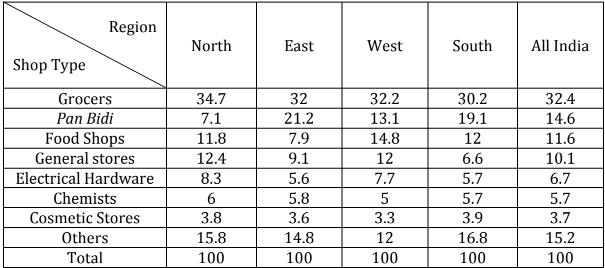All figures are in percentage

Based on survey of ‘shop types’ Kamath categorized Indian states into four geographical regions as shown in the table above. His boss felt that the categorization was inadequate since important labels were missing. Kamath argued that no further labels are required to interpret the data.

Q. A consultant observing the data made the following two inferences:

Inference I: The number of Grocers per-thousand-population is the highest in North India.

Inference II: The number of Cosmetic per-thousand-population is the highest in South India.

Which of following options is DEFINITELY correct?

Solution: Since no data is given on population as well as distribution of states into four zones, it is not possible to calculate per thousand numbers.

QUESTION: 28

Study the data given in the table below and answer the question that follow:All figures are in percentage

Based on survey of ‘shop types’ Kamath categorized Indian states into four geographical regions as shown in the table above. His boss felt that the categorization was inadequate since important labels were missing. Kamath argued that no further labels are required to interpret the data.

Q. The average size of Food Shops in East India was twice that of Food Shops in West India.Which of the following cannot be inferred from the above data?

Solution: Option A and B can be one of the possible conclusions (there are many others) as average size of food shops in East India is higher.

Option D also is a possible conclusion as percentage given is 14.8, which can be converted into 740 (14.8 × 50) or a similar multiple of 14.8

Similarly Option E can also be a possible conclusion since 240 is a multiple of 12 (12 × 20 = 240).

To conclude option C, we require additional data about number of customers and hence cannot be concluded on the basis of data above.

QUESTION: 29

Study the data given in the table below and answer the question that follow:All figures are in percentage

Based on survey of ‘shop types’ Kamath categorized Indian states into four geographical regions as shown in the table above. His boss felt that the categorization was inadequate since important labels were missing. Kamath argued that no further labels are required to interpret the data.

Bala collected the same data five years after Kamath, using the same categorization. His data is presented below: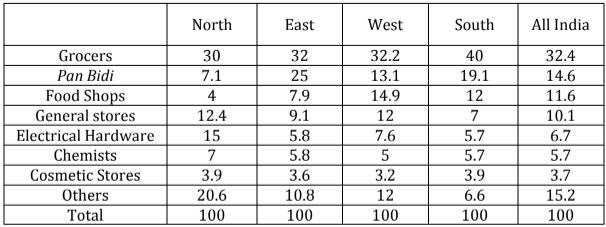Which of the following statements can DEFINITELY be concluded?

Solution: Option A cannot be concluded as there is no mention of gross number in the two time periods.

Using a similar rationale, Option B, C and D cannot be concluded.

Option E can be concluded as population for Pan Bidi shops and Grocers is the same. These are percentages of the same base (total number of shops in East India)